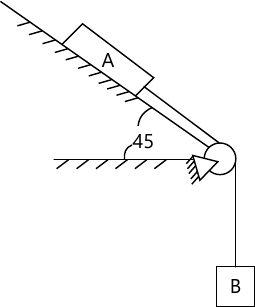# Engineering Mechanics Question paper December 2014

Engineering Mechanics Question paper December 2014 – The first year engineering mechanics paper for mumbai university students of F.E is given as:

### Engineering Mechanics Question paper December 2014

1(a) Four concurrent forces act at a point as shown. Find their resultant.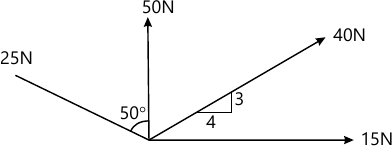4 M
1(b) Define Angle of friction and Angle of repose. Show that Angle of friction is equal to the Angle of repose.
4 M
1(c) A cylinder of weight 500N is kept on two inclined planes as shown in the figure. Determine the reactions at the contact points A & B.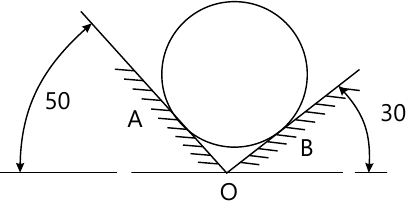4 M
1(d) Acceleration of a particle moving along a straight line is represented by the relation a=30-4.5x2m/s2. The starts with zzero initial velocity at x=0. Determine (a) the velocity when x=3m (b) the position when the velocity is again zero (c) the position when the velocity is maximum.
4 M
1(e) A block of mass 5kg is released from rest along a 40 degree inclined plane. Determine the acceleration of the block using D’Alemberts priniciple. Take coefficient of friction as 0.2.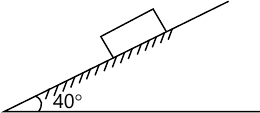4 M
2(a) For given system find resultant and its point of application with respect to point O on the X-axis (X intercept). Force, along CA=100N, along OD=250N, along ED=150N, along OE=100N. A clockwise moment of 5000N-cm is also acting at the point O.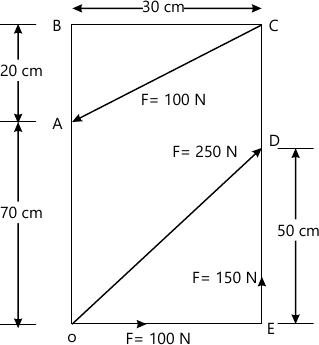6 M
2(b) A cylinder of weight 300N is held in equilibrium as shown in figure given below. Determine the tension in the strong AD and reaction at C & B. The length of AE=750mm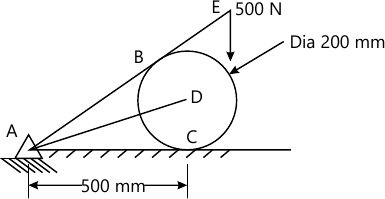8 M
2(c) If a ball is thrown vertically down with a velocity of 10m/s from a height of 3m. Find the maximum height it can reach after hitting the floor, it the coefficient of restitution is 0.7
6 M
3(a) Find centroid of shaded area.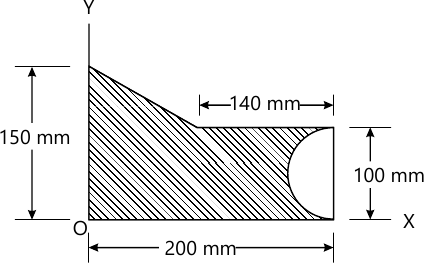8 M
3(b) A rectangular parallelepiped carries three forces shown in figure. Reduce the force system to a resultant force applied at the origin and a moment around origin.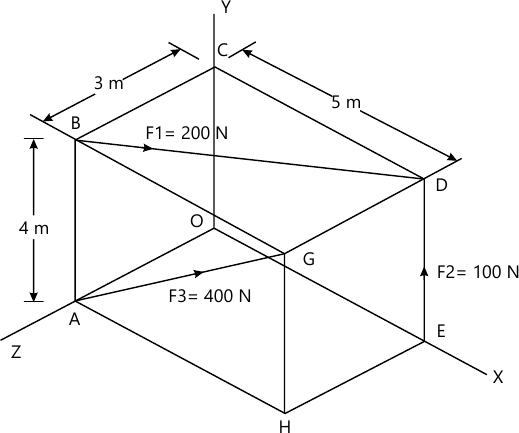6 M
3(c) A collar of mass 1kg is attached to a spring and slides without frinction along a circular rod which lies in a horizontal plane. The spring is undeformed when the collar is at B. Knowing that the collar is passing through the point D with a speed of 1.8m/s, determine the speed of the collar when it passes through point C & B. Take stiffness of the spring, k=250N/m, radius of the circular path=300,, and distance OA=125mm.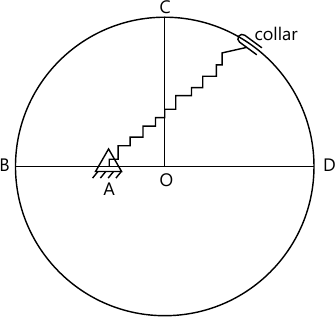6 M
4(a) Find the reactions at supports B & F for the beam loaded as shown in the figure below.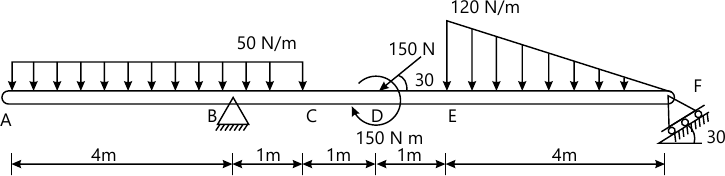8 M
4(b) A particle is projected from the top of a tower of height 50m with a velocity of 20m/s at an angle 30 degrees to the horizontal. Determine:

1. Horizontal distance AB it travel from the foot of the tower.
2. The velocity with which it strikes the ground at B.
3. Total time taken to reach point B.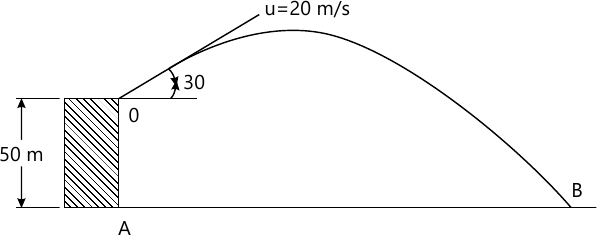6 M
4(c) Figure shows the crank and connecting rod mechanism. The crank AB rotates with an angular velocity of 2 rad/sec in clockwise direction. Determine the angular velocity of connecting rod BC and the velocity of piston C using ICR method. AB = 0.3m and CB = 0.8m.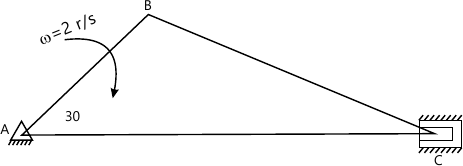6 M
5(a) A truss is loaded as shown in the diagram given below. Determine:

1. Support Reactions
2. Forces on BC, BD by methods of sections.
3. Forces on AB, AE and BE by method of joints.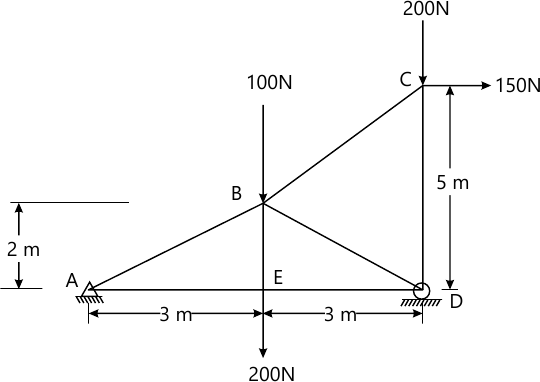8 M
5(b) A particle is projected with an initial velocity of 2m/s along a straight line. The relation between acceleration and time is given in the diagram. Draw v-t and s-t diagram.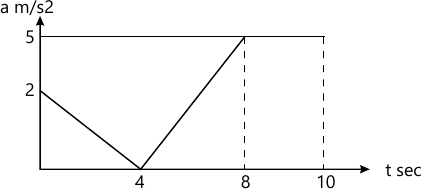6 M
5(c) A wheel of 2m diameter rolls without slipping on a flat surface. The centre of the wheel is moving with a velocity 4m/s towards the right. Determine the angular velocity of the wheel and velocity of points P, Q and R on the wheel.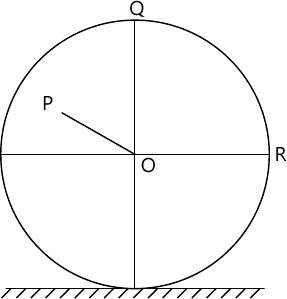6 M
6(a) A force of 100N acts at a point P(-2,3,5)m has its line of action passing through Q(10,3,4)m. Calculate moment of this force about origin (0,0,0).
4 M
6(b) A ladder AB of length 3m and weight 25kg is resting against a vertical wall and a horizontal floor. The ladder makes an angle 50 degree with the floor. A man of weight 60 kg tries to climb the ladder. How much distance along the ladder will he be able to climb if the coefficient of friction between ladder and floor as 0.2 and that between ladder and wall is 0.3. Also find the angle the ladder should make with the horizontal such that the man can climb till the top of the ladder.
8 M
6(c) A particle moves along a track which has a parabolic shape with a constant speed of 10m/s. The curve is given by y=5+0.3x2. Find the components of velocity and normal acceleration when x=2m.
4 M
6(d) Two blocks A & B connected as shown in the diagram. The string is inextensible. Mass of A and B are 3kg and 5kg respectively. If the coefficient of friction between A and inclined plane is 0.25, determine the tension on the strings and accelerations of A & B.